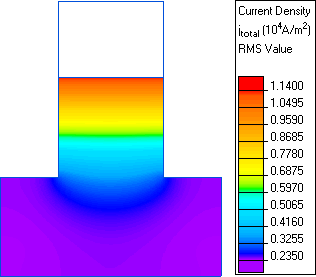QuickField

A new approach to field modelling
 Language: Global English Deutch Espanol Francais Italiano Danmark Ceske Chinese Pycckuü

>> >>

# Slot embedded conductor

This is an example of the slot embedded conductor simulation, performed with QuickField software. A solid copper conductor embedded in the slot of an electric machine carries a current I at a frequency f.

Problem Type:
Plane problem of AC magnetics.

Geometry:

Given:
Magnetic permeability of air μ = 1;
Magnetic permeability of copper μ = 1;
Conductivity of copper σ = 58,005,000 S/m;
Current in the conductor I = 1 A;
Frequency f = 45 Hz.

Problem:
Determine current distribution within the conductor and complex impedance of the conductor.

Solution:
We assume that the steel slot is infinitely permeable and may be replaced with a Neumann boundary condition. We also assume that the flux is contained within the slot, so we can put a Dirichlet boundary condition along the top of the slot. See model file for the complete model.

The complex impedance per unit length of the conductor can be obtained from the equation

Z = V / I

where V is a voltage drop per unit length. This voltage drop on the conductor can be obtained in Local Values mode of the postprocessing window, clicking an arbitrary point within the conductor.

Results:
Current density in slot embedded conductor:Re Z (Ohm/m) Im Z (Ohm/m) Reference* 0.00017555 0.00047113 QuickField 0.00017550 0.00047111

* Reference: A. Konrad, Integrodifferential Finite Element Formulation of Two-Dimensional Steady-State Skin Effect Problems, IEEE Trans. Magnetics, Vol MAG-18, No. 1, January 1982.

• View simulation report in PDF
• Download simulation files (files may be viewed using any QuickField Edition).